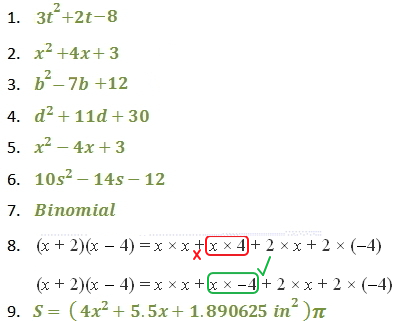Multiply Binomials

Multiply Binomials

You can use a visual model to multiply binomials. The model below shows that the product (x + 2)(3x + 1) equals

 x x x 1 x x2 x2 x2 x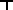x + 2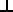1 x x x 1 1 x x x 1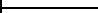3x + 1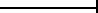the solution for the binomial above is

3x2 + 7x +2

You can multiply two binomials using a table or a vertical method,

Multiplying Binomials with a Table

Example

Find the product (-3x + 2)(8x + 7) and simplify.

Write the first polynomials on the left of the table and the second polynomial above the table

 8x 7 -3x -24x2 -21x 2 16x 14

Multiply to fill the table

Combine like terms

-24x2 - 21x + 16x + 14

-24x2 - 5x + 14

The answer is: -24x2 - 5x + 14

Multiplying Binomials Vertically

Example

Simplify the following expression: (2x - 5)(3x +  4)

Solution

Write and line up the first and second binomial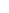2x  -  5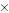3x  + 4

Multiply 4(2x - 5)2x  -  53x  + 48x  -  20

Multiply 3x(2x - 5) and line up like terms2x  -  53x  + 48x  -  20

6x2 - 15x

Add 8x - 20 and 6x2 - 15x2x  -  53x  + 48x  20

6x2 - 15x

6x2 - 7x - 20

The result is  6x2 - 7x - 20

Multiplying Binomials Horizontally

Example

You deposit \$1 into a savings account with interest compounded annually. The balance of the account after two years can be found using the expression (1 + r)2, where r represents the interest rate. Expand this expression and simplify.

Solution

To expand the expression, multiply 2 binomials.

Expand (1 + r)2

(1 + r)2 = (1 + r)(1 + r)

then use the distributive property

(1 + r)(1 + r) = 1(1 + r) + r(1 + r) = 1 + r + r + r2

Simplify and write in standard form

1 + r + r + r2 = 1 + 2r + r2 = r2 + 2r + 1

Try it yourself

Find the product and simplify.

1.) (3t - 4)(t + 2)

2.) (x + 1)(x + 3)

3.) (b - 4)(b - 3)

The FOIL Method

The letters in the word FOIL can help you remember how to multiply binomials. The letters should remind you of the words

First, Outer, Inner, Last

F         L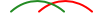(a + b) (c + d)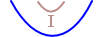O

Multiplying with the FOIL Method

Find the product (2x + 3)(3x - 1) and simplify.

 F O I L First + Outer + Inner + Last 2x3x + 2x(-1) + 33x + 3(-1) Group terms 6x2 + (-2x) + 9x + (-3) Multiply 6x2 + 7x - 3 Combine like terms

Try it yourself

Find the product and simplify.

4.) (d + 6)(d + 5)

5.) (x - 3)(x - 1)

6.) (5s + 3)(2s - 4)

7.) Complete the sentence.
A polynomial with two terms is called a _?_.

8.) Describe and correct the error in the solution.

(x + 2)(x - 4) = (xx) + (x-4) + (2x) + (2(-4))

(x + 2)(x - 4) = (xx) + (x-4) + (2x) + (2(-4))

(x + 2)(x - 4) = x2 + 4x + 2x - 8

(x + 2)(x - 4) = x2 + 6x - 8

9.)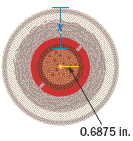The middle of a baseball is a cork sphere with a radius of 0.6875 inches, and x is the thickness of the rubber and yarn coating surrounding the cork.

Use the formula S = 4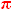r2 and write a polynomial expression for the surface area of the baseball. Expand the expression and simplify.